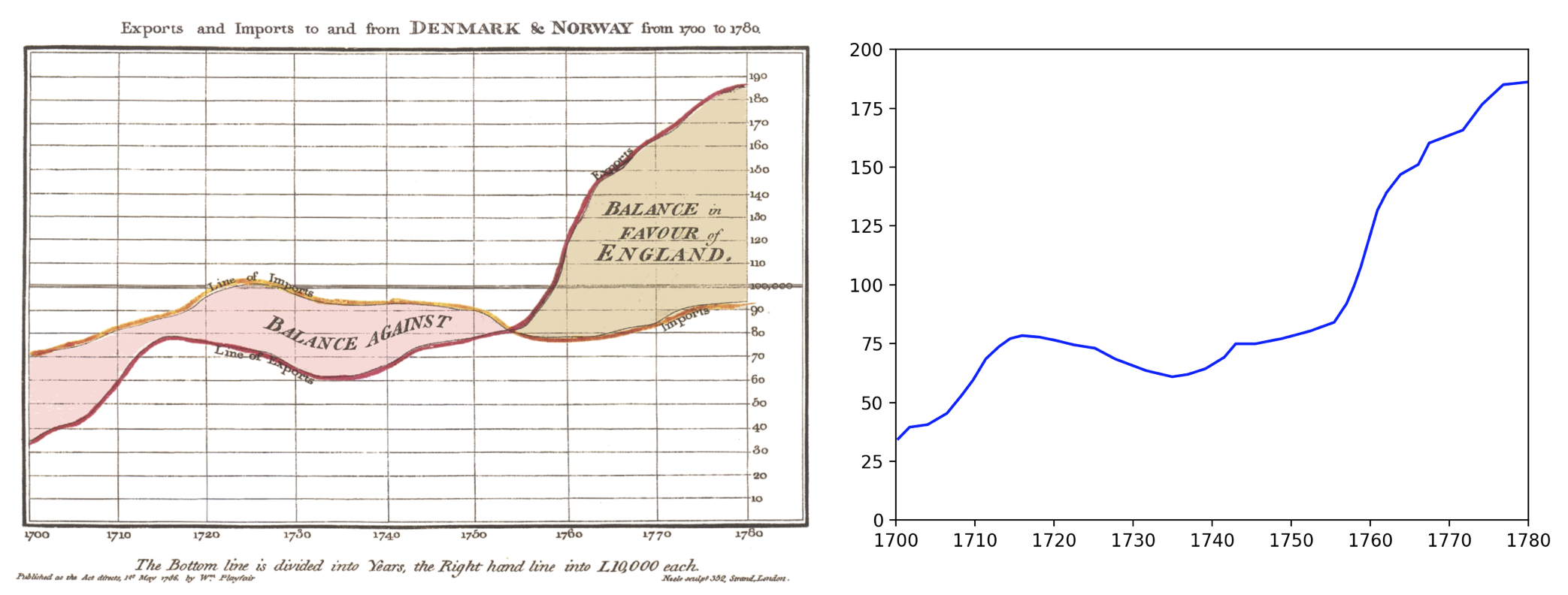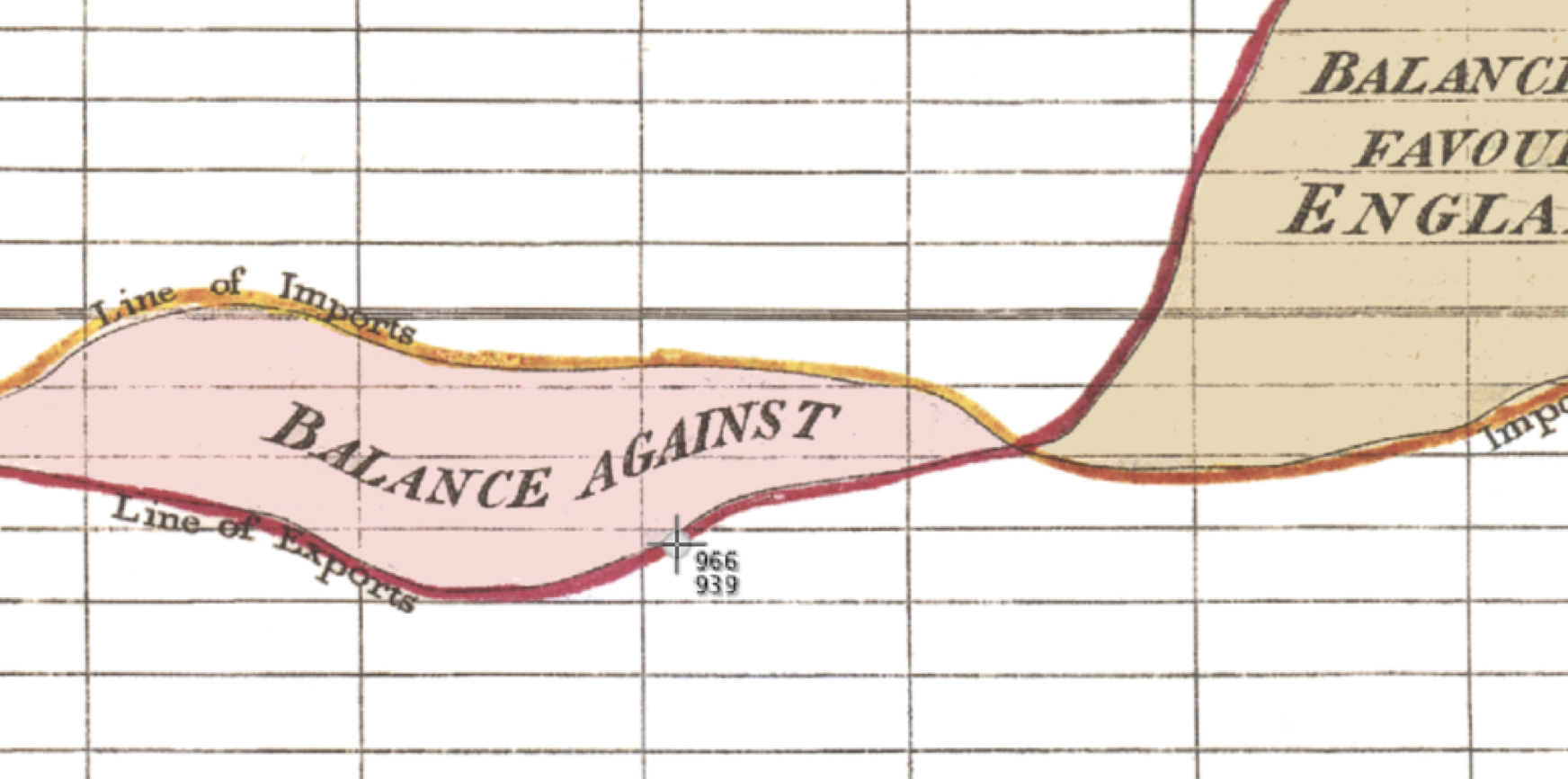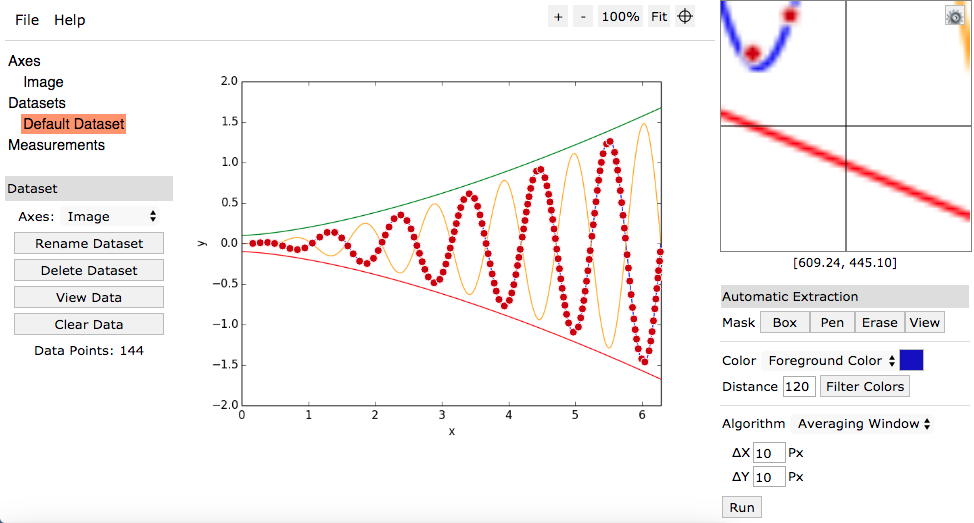# Digitize a GraphI recently needed to extract data from the screenshot of a graph. The data was provided by a third party in that format so I had to work with what I was given.

## Extract with Mac Screen Coordinates

A quick way to extract the data is to use the Mac screen capture tool to display the screen coordinates of the cursor. For this example I will use the chart "Exports and Imports to and from Denmark & Norway from 1700 to 1780" by William Playfair1.You can drag the cursor across the line, write down the screen coordinates at various intervals, and recreate the chart. Note that the origin of the screen coordinates is the top left and the units are in pixels. Therefore you will need to invert the y-axis and scale to fit the origin of the graph.

This gets the job done, but is certainly not ideal (or quick).

## Extract with Python

Next I decided to write a short Python Script to extract and convert the coordinates by clicking on them. First you select the origin and top right corner to scale the axes, and then click through some points to capture the curve. You need to type in the x- and y-scale, and then it scales the data from pixes to the units given.

from pynput import mouse

class MyException(Exception):pass

X = []
Y = []
NumberOfMouseClicks = 0
print('Click Origin')

def on_click(x, y, button, pressed):
button = str(button)
global NumberOfMouseClicks

NumberOfMouseClicks = NumberOfMouseClicks + 1
if NumberOfMouseClicks==1:
print('Click Top Right')
if NumberOfMouseClicks==3:
print('Click data points. Right-click to end.')

X.append(x)
Y.append(y)

if button!='Button.left':
raise MyException(button)

def plot_data(X, Y, Xmin, Xmax, Ymin, Ymax):
import matplotlib.pyplot as plt

plt.plot(X,Y,'b-')
plt.xlim((Xmin, Xmax))
plt.ylim((Ymin, Ymax))
plt.show()

def main(X,Y):
with mouse.Listener(on_click=on_click) as listener:
try:
listener.join()
except MyException as e:
pass

# drop duplicates
X = X[::2]
Y = Y[::2]

# input boundaries
Xmin = float(input('Input X-min: '))
Xmax = float(input('Input X-max: '))
Ymin = float(input('Input Y-min: '))
Ymax = float(input('Input Y-max: '))

# define scales from data
origin = [X,Y]
topRight = [X,Y]
XminScale = origin
XmaxScale = topRight
YminScale = origin
YmaxScale = topRight

# drop extras
X = X[2:-1]
Y = Y[2:-1]

# scale
## (old_value - old_min) / (old_max - old_min) * (new_max - new_min) + new_min
Xplot = [(i - XminScale) / (XmaxScale - XminScale) * (Xmax - Xmin) + Xmin for i in X]
Yplot = [(i - YminScale) / (YmaxScale - YminScale) * (Ymax - Ymin) + Ymin for i in Y]

# print outputs
print('Origin:     {}'.format([round(i, 2) for i in origin]))
print('Top Right:  {}'.format([round(i, 2) for i in topRight]))
print('X: {}'.format([round(i, 2) for i in Xplot]))
print('Y: {}'.format([round(i, 2) for i in Yplot]))

# plot
plot_data(Xplot, Yplot, Xmin, Xmax, Ymin, Ymax)

if __name__ == '__main__':
main(X,Y)


Here it is in action:And here is the result:## WebPlotDigitizer

A few days after doing the above I came across the WebPlotDigitizer prjoect by Ankit Rohatgi. This is a much more sophistocated tool for extracting data from images that includes a web app, support for multiple types of plots, and algorithms for automatically extracting data.In a talk at PLOTCON 2017 Ankit demonstrated the tool and its many features.

I plan to use WebPlotDigitizer in the future when I come across a similar problem, but it was fun figuring out the basics in Python.

1. Edward Tufte, The Visual Display of Quantitative Information, (New York: Graphics Press), 92.

© 2005 Matthew Kudija | Source